## Archive for the 'magnetism' Category

27
Oct
07

### Magnetic Pendulum Strange Attractor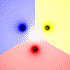This chaotic strange attractor represent the final resting positions for a magnetic pendulum suspended over some magnets (shown as black dots). It kind of looks like mixed paint. The 2D animation shows what happens as you decrease the damping factor. The 3D animation was shaded by path length.

Mathematica 4.2 version: 1/24/05; C++ version: 10/27/07; 3D rendered in POV-Ray 3.1

```(* runtime: 25 seconds, increase n for higher resolution *) n = 40; h = 0.25; g = 0.2; mu = 0.07; zlist = {Sqrt + I, -Sqrt + I, -2I}; image = Table[z2 = z /. NDSolve[{z''[t] == Plus @@ ((zlist - z[t])/(h^2 + Abs[zlist - z[t]]^2)^1.5) - g z[t] - mu z'[t], z == x + I y, z' == 0}, z, {t, 0, 25}, MaxSteps -> 200000][]; r = Abs[z2 - zlist]; i = Position[r, Min[r]][[1, 1]]; Hue[i/3], {y, -5.0, 5.0, 10.0/n}, {x, -5.0,5.0, 10.0/n}]; Show[Graphics[RasterArray[image]], AspectRatio -> 1];```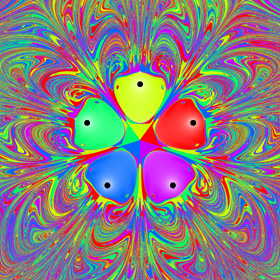The picture on the left shows another version with five magnets. See also my physics pendulums.

Here is some Mathematica code to numerically solve this using the Beeman integration scheme with the predictor-corrector modification:
```(* runtime: 45 seconds, increase n for higher resolution *) n = 40; tmax = 25; dt = 0.1; h = 0.25; g = 0.2; mu = 0.07; zlist = {Sqrt + I, -Sqrt + I, -2I}; image = Table[z = x + I y; v = a = a1 = 0; Do[z += v dt + (4a - a1)dt^2/6; vpredict = v + (3a - a1)dt/2; a2 = Plus @@ ((zlist - z)/(h^2 + Abs[zlist - z]^2)^1.5) - g z - mu vpredict; v += (2a2 + 5a - a1)dt/6; a1 =a; a = a2, {t, 0, tmax, dt}]; r = Abs[z - zlist]; Hue[Position[r, Min[r]][[1, 1]]/3], {y, -5.0, 5.0, 10.0/n}, {x, -5.0, 5.0, 10.0/n}]; Show[Graphics[RasterArray[image]], AspectRatio -> 1];```

Advertisements
01
Oct
06

### 3-Phase Brushless DC (BLDC) MotorBrushless motors are popular in model airplanes. This one has a 6 pole stator and an 8 pole rotor. The technique used here is very similar to that used in Maglev trains. See also my Tesla coil magnetic field.

15
Jul
06

### Horseshoe Magnets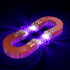These magnetic fields were made using the same technique as the solenoid picture.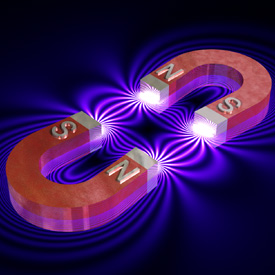new version: POV-Ray 3.6.1, 7/15/06
old version: calculated in Mathematica 4.2, rendered in TrueSpace 4.3, 3/9/03

17
May
06

### Magnetic Field of a Solenoid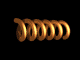This magnetic field was approximated by a superposition of 2D point sources using the Biot-Savart Law. You can also see the old version of this picture on Jeff Bryant’s Mathematica visualization site. Click here to download the POV-Ray code for this image. See also my magnetic field representations for a motor, Tesla coil, and horseshoe magnets.

new version: POV-Ray 3.6.1, 5/17/06; old version: calculated in Mathematica 4.2, rendered in TrueSpace 4.3, 1/19/03

```(* runtime: 12 seconds *) plist = Table[{(4 i - 26)/6, -(-1)^i}, {i, 1, 12}]; r[{xi_, yi_}] := Sqrt[(x - xi)^2 + (y - yi)^2]; DensityPlot[2Sqrt[((Plus @@ Map[#[](x - #[])/r[#]^2 &, plist])^2 + (Plus @@ Map[-#[](y - #[])/r[#]^2 &, plist])^2)] + Cos[18.8Plus @@ Map[#[]/r[#] &, plist]] + 1, {x, -6, 6}, {y, -3, 3}, Mesh -> False, Frame -> False, PlotRange -> {0, 10}, PlotPoints -> {275, 138}, AspectRatio -> 1/2]```

07
Apr
06

### Magnet Fractals

These fractals were originally designed for predicting magnetic phase-transitions.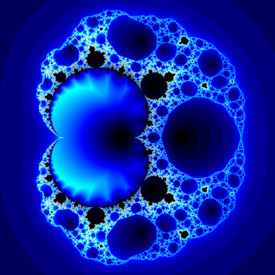Here is some Mathematica code:
```Mandelbrot[zc_] := Length[FixedPointList[f[#, zc] &, 0, 100, SameTest -> (Abs[#] > 2 &)]]; Magnet[] := DensityPlot[Log[Mandelbrot[xc + I yc]], {xc, -1, 3}, {yc, -2, 2}, PlotPoints -> 275, Mesh -> False, Frame -> False, ColorFunction -> (If[# != 1, Hue[#], RGBColor[0, 0, 0]] &)];```

``` (* Magnet 1: runtime: 5 minutes *) f[z_, zc_] := (z^2 + zc - 1.0)^2 / (2 z + zc - 2)^2; Magnet[]; ```

```(* Magnet 2: runtime: 15 minutes *) f[z_, zc_] := (z^3 + 3 (zc - 1) z + (zc - 1) (zc - 2))^2 / (3 z^2 + 3 (zc - 2) z + (zc - 1) (zc - 2) + 1.0)^2; Magnet[]; ```
POV-Ray has a built-in function for these fractals:
```//Magnet 1: runtime: 0.5 second camera{orthographic location <1.25,0,-2> look_at <1.25,0,0> angle 90} plane{z,0 pigment{magnet 1 mandel 50 interior 1,1 color_map{[0 rgb 0][1/6 rgb <0,0,1>][1/3 rgb 1]}} finish{ambient 1}}```

``` ```

```//Magnet 2: runtime: 0 seconds camera{orthographic location <1,0,-2> look_at <1,0,0> angle 90} plane{z,0 pigment{magnet 2 mandel 50 color_map{[0 rgb 0][1/6 rgb <1,0,0>][1/3 rgb 1][1 rgb 1][1 rgb 0]}} finish{ambient 1}}```

## Welcome !

You will find here some of my favorite hobbies and interests, especially science and art.

I hope you enjoy it!

Subscribe to theRSS feed to stay informed when I publish something new here.

I would love to hear from you! Please feel free to send me an email : bugman123-at-gmail-dot-com

## Recent CommentsBerna Blalack on Magnetic Pendulum Strange…Daan on Magnetic Pendulum Strange…Sebastian Schepis on Diffusion Limited Aggregation…mohammad_andito on CFM56-5 Turbofan Jet Engi…SasQ on Magnetic Field of a Solen… OUPblog » Blog… on Diamond Light Dispersion Complex Roots on Polynomial Roots Joukowski airfoils |… on Joukowski AirfoilKarim Alame on Flapping WingREJISH J on Joukowski Airfoil SOLINOID | Materials… on Magnetic Field of a Solen…Emanuele on 4D “Squarry” Julia…Emanuele on Hydrogen Electron Orbital Prob…Tim on Mandelbrot Set Pickover S…khankasi1 on 4D Quaternion Mandelbrot …

• 561,072 hits
Advertisements